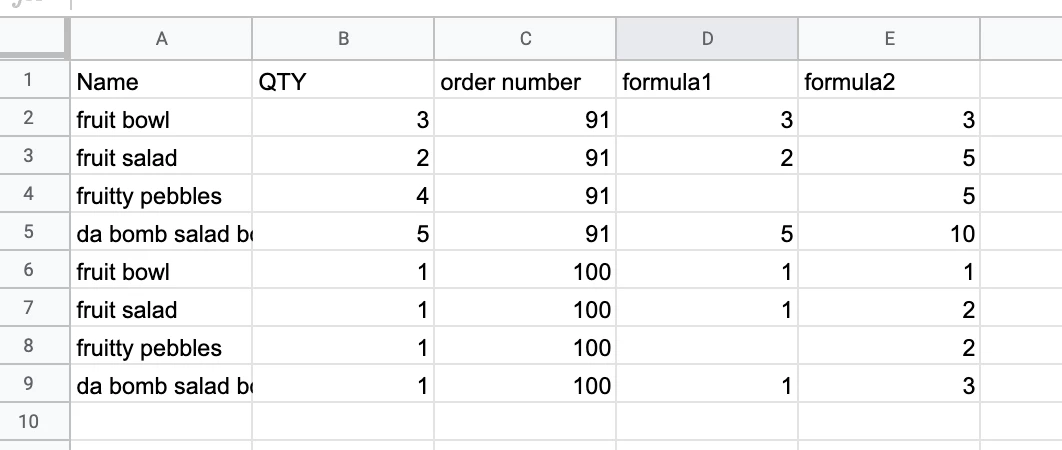# Extracting and combining data from shopify order

• 3 replies
• 239 views

Userlevel 1
• drucki• Beginner
• 2 replies

Hello! I have recorded a short 1min video explaining what I am trying to do.

https://www.loom.com/share/1cd34826fba64731a456bd14ba3df5d2

Deep THANK YOU to whoever can help me with this. Thank you so much :)

Derek

icon

Best answer by PaulKortman 28 July 2020, 01:38

View original

### 3 replies

Userlevel 7+10
• PaulKortman• Certified Zapier Expert
• 468 replies

@drucki this can be done with a temporary Google Sheet,

so I’d set up a google sheet to have 4 columns, Name, Qty, Order #, Formula1, and Formula2

I would have a step in zapier Google Sheets > Create new row(s) and put the line items name in the first column, the line items quantity in the second column and the order # in the third column. in the fourth column (Formula1) I would put the following formula

``=if(find("salad","{{line item name}}")>0,{{line item qty}},if(find("bowl","{{line item name}}")>0,{{line item qty}},""))``

Note: I’ve never tried this with line items in a formula like this so that formula might not work, if not see the alternative formula option below.

Then I would add an additional step that find multiple rows where the Order # = the order number of this order (from shopify). You will then have the Quantities you need to add up in the Formula column.

Alternative Formula Idea:

Instead of writing the formula using Zapier, you could use the following which is Google sheet’s methodology and copy it down from row 2 to the 1000th row in the sheet - not ideal because it will eventually run out.

``=if(iferror(find("salad",A2),false),B2,if(iferror(find("bowl",A2),false),B2,""))``

you could then add the following formula to the 5th column and copy this down

``=if(c1=c2,e1+d2,d2)``

this formula2 basically reads, if the order number of this row (c2) equals the order number of the row above (c1) then add the formula1 of this row (d2) to the formula2 of the row above (e1). If this row’s order number does not equal the row’s order number above it then just put the results of this row (d2).

Then you can change the search step in Zapier Google Sheets to just look for one row and search from the bottom up, the last row in any given order number in the e column (formula2) will have the total number of salads and bowls.

Sometimes it’s easier to see it in action:By searching from the bottom up for order number 91 column E will give you 10 as the result, similarly order number 100 will give 3 as the result

Userlevel 1
• drucki• Author
• Beginner
• 2 replies

@PaulKortman Thank you so much again for the incredibly thoughtful response. I am having some difficulty getting this to work after trying both approaches.

I have described what I think is the main problem in this screen recording if you think there is an easy solution I would love to hear it :)

https://www.loom.com/share/c65668b06fca44ee97e67f719e9bd5ab

Userlevel 7+10
• PaulKortman• Certified Zapier Expert
• 468 replies

@drucki you’re so close! I just want to make sure the zap step that you have for creating new rows in Google Sheets is actually the Create Spreadsheet Row(s) and not the Create a Spreadsheet Row

If that is the action step you are using and it’s still not putting multiple lines into the Google Sheet you might need to run those two values through the Formatter > Utilities > Text to Line Items as seen below. and then use the output of these two steps (one for each the name and the quantity)  in the Google Sheets create rows step.